# C Program to Generate Prime Factor

Definition of Prime Factor from Wikipedia: In number theory, the prime factors of a positive integer are the prime numbers that divide that integer exactly. The process of finding these numbers is called integer factorization, or prime factorization.

Example: Prime factors of 288 are:
288 = 2 x 2 x 2 x 2 x 2 x 3 x 3

Here is a you tube video for you that will help you to understand prime factorization.

Now we are moving towards a C program that will find the prime factors of a number.

#### C Program to Find Prime Factors:

```#include <stdio.h>

int main()
{

int number,div;
printf("Enter a number to know its prime factor: ");
scanf("%d",&number);

printf("\nThe prime factors of %d are: \n\n",number);

div = 2;

while(number!=0){
if(number%div!=0)
div = div + 1;
else {
number = number / div;
printf("%d ",div);
if(number==1)
break;
}
}
return 0;
}
```
Share:

#### 10 comments :

1.nice programming, Can you please provide links where I can hone my programming skills as a beginner.

1.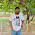http://www.mycodeschool.com/ is a good site for beginners.

2.Best Method... (h)

3.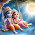4.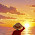5.Thanks for nice post.C programming details here

6.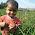Can you pls xplain d program prime factor

7.this coding is Nice.

8.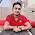Prime number program in C++

A prime number is a natural number which is greater than 1 and these numbers are only divisible by 1 and itself number. In programming point of view, we check condition number is divisible by other number or not except 1 and number itself. If number is not divisible by other number than that number is prime number.

9.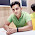Prime number program in C

Thanks for this post, keep sharing

Spam comments will be deleted. :)